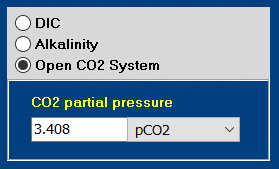# Pristine Rainwater

What is the chemical composition of pristine rainwater?

Preliminary Consideration

‘Pristine rainwater’ is pure water in chemical equilibrium with the CO2 gas of the atmosphere (no other acids and bases):

 (1) pristine rainwater   =   H2O + CO2(g)

It is an example par excellence of the open carbonate system. The partial pressure of CO2(g) is 0.00039 atm. In hydrochemistry, it is quite common to express the partial pressure by the pCO2 value:1

 (2) pCO2  =  – log P  =  – log 0.00039  =  3.408Calculation and Results

We start with pure water (button H2O) and select “Open CO2 System” – as shown in the right screenshot. It displays the default value pCO2 = 3.408, which represents the partial pressure of CO2 in the atmosphere. [It is easy to check it: Select in the blue drop-down list “atm”, then 0.0039 atm appears.]

Click on Start to run the calculations. The first information you get is that pH changes from 7 to 5.61. The complete results are shown in the output tables (including table Ions) for 25.

 pH =   5.61 DIC =   0.0157 mM (= 0.189 mg/L) CO2 =   0.0133 mM HCO3- =   0.0024 mM CO3-2 =   4.7 · 10-8 mM

Here, CO2 represents the composite carbonic acid H2CO3*. The sum of CO2 and HCO3- yields the DIC. In this slightly acidic water there is virtually no carbonate ion, CO3-2.

Remarks & Footnotes

1. Many textbooks use the (rounded) pCO2 value of 3.5. So, one can rerun the above calculations with pCO2 = 3.5, if desired. What we then obtain is: pH 5.66 and DIC = 0.0130 mM.